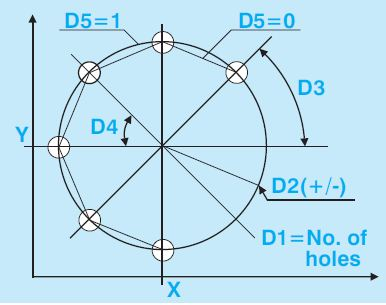# Selca Circumference pattern drilling

Selca Circumference pattern drilling

## Parameters

ParameterDescription
X,Ycircumference center coordinates.
D1=number of holes.
D2=circumference radius with sign. If the radius is positive, holes will be machined CCW; if the radius is negative, they will be machined CW.
D3=angle formed by the first hole and the X-axis (D3=0° by default).
D4=angular distance between holes (if D4 is omitted, figures will be evenly distributed over 360°).
D5=specifies the displacement mode between 2 consecutive holes:
D5=0 linear displacement on the clearance plane (default value)
D5=1 circular displacement on the clearance plane.